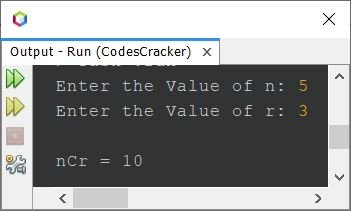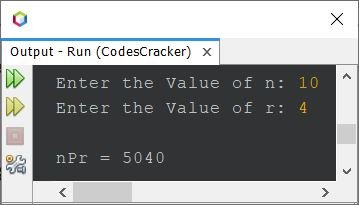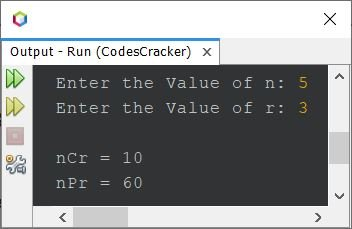# Java Program to Find nCr and nPr

This article is created to cover multiple programs in Java that find and prints nCr (Combination) and nPr (Permutation) based on the n and r value entered by user at run-time of the program.

The formula to find the value of nCr (Combination) is:

`nCr = n!/r!(n-r)!`

In above formula, ! is the symbol of factorial. Factorial of a number n is calculated as n * (n-1) * (n-2) * (n-3) * ... * 1.

The formula to find the value of nPr (Permutation) is:

`nPr = n!/(n-r)!`

Note - nCr value shows the number of ways to select r things out of n.

Note - nPr value shows the number of ways to arrange r things out of n.

## Find nCr (Combination) in Java

The question is, write a Java program to find nCr. The n and r value must be received by user at run-time. The program given below is its answer:

```import java.util.Scanner;

public class CodesCracker
{
public static void main(String[] args)
{
int n, r, ncr, fact=1, numerator, denominator, i=1, sub;
Scanner s = new Scanner(System.in);

System.out.print("Enter the Value of n: ");
n = s.nextInt();
System.out.print("Enter the Value of r: ");
r = s.nextInt();

while(i<=n)
{
fact = fact*i;
i++;
}
numerator = fact;       // n!
sub = n-r;
fact = 1;
i = 1;
while(i<=sub)
{
fact = fact*i;
i++;
}
denominator = fact;     // (n-r)!
fact = 1;
i = 1;
while(i<=r)
{
fact = fact*i;
i++;
}
denominator = (fact*denominator);    // r!(n-r)!
ncr = numerator/denominator;

System.out.println("\nnCr = " +ncr);
}
}```

The sample run of above program with user input 5 as value of n, and 3 as value of r, is shown in the snapshot given below:Since nCr value is 10 as shown in the above output. Therefore, there are 10 ways to select 3 things out of 5.

### Find nCr in Java using Function

Previous program provides the step by step calculation of nCr value. Now let's shorten the program. This program uses user-defined function to keep the program as short as possible.

```import java.util.Scanner;

public class CodesCracker
{
public static void main(String[] args)
{
int n, r, ncr;
Scanner s = new Scanner(System.in);

System.out.print("Enter the Value of n: ");
n = s.nextInt();
System.out.print("Enter the Value of r: ");
r = s.nextInt();

ncr = (fact(n)) / (fact(r)*fact(n-r));

System.out.println("\nnCr = " +ncr);
}
public static int fact(int num)
{
int fact=1;
for(int i=1; i<=num; i++)
fact *= i;
return fact;
}
}```

That's the use of function. See the code becomes too short, when created using function. Because in first program, many times, the same code of finding factorial are used again and again. But now using function, the code is created only at once and can be used for any number of times.

## Find nPr (Permutation) in Java

This program find and prints the nPr value. This program too uses function to do the job.

```import java.util.Scanner;

public class CodesCracker
{
public static void main(String[] args)
{
int n, r, npr;
Scanner s = new Scanner(System.in);

System.out.print("Enter the Value of n: ");
n = s.nextInt();
System.out.print("Enter the Value of r: ");
r = s.nextInt();

npr = (fact(n)) / (fact(n-r));

System.out.println("\nnPr = " +npr);
}
public static int fact(int num)
{
int fact=1;
for(int i=1; i<=num; i++)
fact *= i;
return fact;
}
}```

Here is its sample run with user input 10 as the value of n and 4 as the value of r:That is, there are 5040 ways to arrange 4 things out of 10.

## Find nCr and nPr in Java

This is basically the combined version of previous programs. Because this program find and prints the value of nCr and nPr both.

```import java.util.Scanner;

public class CodesCracker
{
public static void main(String[] args)
{
int n, r, ncr, npr;
Scanner s = new Scanner(System.in);

System.out.print("Enter the Value of n: ");
n = s.nextInt();
System.out.print("Enter the Value of r: ");
r = s.nextInt();

npr = (fact(n)) / (fact(n-r));
ncr = npr/fact(r);

System.out.println("\nnCr = " +ncr);
System.out.println("nPr = " +npr);
}
public static int fact(int num)
{
int fact=1;
for(int i=1; i<=num; i++)
fact *= i;
return fact;
}
}```

The snapshot given below shows the sample run with user input 5 and 3 as value of n and r:#### Same Program in Other Languages

Java Online Test

« Previous Program Next Program »